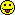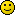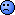☆

2008-03-20 12:33
(5671 d 05:45 ago)

Posting: # 1714
Views: 12,514

## Intersubject variation in replicated BE study [General Sta­tis­tics]

Dear HS/all,

Please suggest wheather we can calculate intersubject variation in replicated four period, two sequence, two formulation bioequivalence study using WinNonlin or SAS software as per the model given in Guidance for Industry Statistical Approaches to Establishing Bioequivalence or any other method to calculate the same.

If yes kindly suggest the method to calculate intersubject variation.

Regards,

Atish
d_labes
★★★

Berlin, Germany,
2008-03-26 14:34
(5665 d 03:44 ago)

Posting: # 1722
Views: 10,403

## Intersubject variation in replicated BE study

Dear Atish,

❝ ... wheather we can calculate intersubject variation in

❝ replicated four period, two sequence, two formulation bioequivalence study

❝ .... SAS software as per the model given in Guidance for

❝ Industry Statistical Approaches to Establishing Bioequivalence ...

The intersubject variation (separated for Test and Reference) is part of the model of the FDA and therefore part of the output of the SAS code given in Appendix E of the guidance.

Look for G-matrix.

forum_entry.php?id=1589
or
forum_entry.php?id=1312
here in the forum and puzzle things together.

Regarding WINNONLIN I am not an initiate.

By the way: Why do you need the intersubject variation?

Regards,

Detlew
☆

2008-03-31 17:02
(5660 d 02:16 ago)

@ d_labes
Posting: # 1731
Views: 10,480

## Intersubject variation in replicated BE study

Dear d_labes,

Thank you for the reply, I need inter-subject variation because it is written in the protocol.
After refering to the mail given to Andrew by Dave and GSK BDS Technical Report 2002 - 01, I found the answer to the question.

❝ The replicate SAS output gives all of the information needed to calculate

❝ intra- and inter-CV, in it outputs of covariate matrix ("Covariate

❝ Parameter Estimates"). The SAS output look like this:

❝ Covariate Parameter Estimates

❝ Cov Parm Subject Group Estimate

❝ FA(1,1) Subject 0.4289 <---- (sig_BR, the between-subject standard

❝ deviation for the Reference product)

❝ FA(2,1) Subject 0.3131 <---- (sig_BT, the between-subject standard

❝ deviation for the Test product)

❝ FA(2,2) Subject <---- (sig_D, the subject-by-formulation interaction

❝ term)

❝ Residual Subject Formulation R 0.1479 <---- (sig_WR^2, the within-subject

❝ standard deviation for the Reference product)

❝ Residual Subject Formulation T 0.2539 <---- (sig_WT^2, the within-subject

❝ standard deviation for the Test product)

❝ The intra-subject variabilities are still calculated from the residuals as

❝ in a 2-way crossover, using:

❝ IntraCV_R = 100%*sqrt(exp(sig_WT^2)-1) (in this case, =

❝ 100*sqrt(exp(0.1479)-1) = 39.92%

❝ IntraCV_T = 100%*sqrt(exp(sig_WR^2)-1) (in this case, =

❝ 100*sqrt(exp(0.2539)-1) = 53.76%

❝ FA(1,1) and FA(2,1) are the inter-subject standard deviations for Reference

❝ and Test products, respectively:

❝ InterCV_R = 100%*sqrt(exp(sig_BT^2)-1) = 100*sqrt(exp(0.4289^2)-1) = 44.94%

❝ InterCV_T = 100%*sqrt(exp(sig_BR^2)-1) = 100*sqrt(exp(0.3131^2)-1) = 32.09%

Kindly let me know whether the above calculate inter and intra subject variability are correct.

In SAS code the Random statement, TYPE=FA0(2) if replaced by TYPE=CSH we could directly get inter and intra subject variance, isn't it?

Regards,

Atish
Jaime_R
★★

Barcelona,
2008-03-31 17:09
(5660 d 02:09 ago)

Posting: # 1733
Views: 10,294

## Intersubject variation in replicated BE study

Dear Atish!

❝ [...] I need inter-subject variation because it is written in the protocol.

                               ^^^^^^^^^^^^^^^^^^^^^^^^^^^^^^
That's a reason!(sorry, but I could not resist)

Regards, Jaime
☆

2008-04-01 08:15
(5659 d 11:04 ago)

@ Jaime_R
Posting: # 1734
Views: 10,635

## Intersubject variation in replicated BE study

Dear d_labes/all,

subject-by-formulation interaction variance is
sig_D^2=FA(1,1)^2+FA(2,1)^2-2*FA(1,1)*FA(2,1)

As far as WinNonlin is concerned Under 'Final Variance Parameters' values of lambda(1,1)_11, lambda(1,2)_11 and lambda(2,2)_11 corresponds to SAS output of FA(1,1), FA(2,1) and FA(2,2) respectively.

Based on above mentioned parameters we can calculate inter and intra subject variation.

Regards,

Atish
d_labes
★★★

Berlin, Germany,
2008-04-01 12:01
(5659 d 07:17 ago)

Posting: # 1737
Views: 10,532

## Intersubject variation in replicated BE study

Dear Atish,

to puzzle is an artand to read needs glasses.

❝ FA(1,1) and FA(2,1) are the inter-subject standard deviations for

❝ Reference and Test products, respectively ...

This is not correct in my opinion. See my last comment in the thread here.
To state it once more:
Apart from the specific model used the G-matrix contains the inter-individual variabilities (diagonal elements).
This matrix can be parameterized in different ways.
Using the FA0(2) parameterization you get:
  sig_BR2=G(1,1)=FA(1,1)2   sig_BT2=G(2,2)=FA(2,1)2+FA(2,2)2

By the way: Be shure your coding of R preceeds T, else change indices.

Check it in comparing to the CSH parameterization in which case you have
(with the SAS-output names of the covariance parameters):
  sig_BR2=G(1,1)=Var(1)   sig_BT2=G(2,2)=Var(2)

❝ InterCV_R = 100%*sqrt(exp(sig_BT^2)-1 ....

❝ InterCV_T = 100%*sqrt(exp(sig_BR^2)-1) ...

Correct only if you use sig_BR in the first formula and sig_BT in the second.
Correct only if you analyze log-transformed PK parameters.

❝ In SAS code the Random statement, TYPE=FA0(2) if replaced by TYPE=CSH we

❝ could directly get inter and intra subject variance, isn't it?

That's correct to me. See above.

Your formula regarding subject-by-formulation interaction later on in this thread (after Jaime's spontaneous outcry) is not correct to me.
It is only valid, if the correlation (thinking in the CSH model) amounts to 1.
In general it reads in the FA0(2) model
 sig_D2=FA(1,1)2+FA(2,1)2+FA(2,2)2-2*FA(1,1)*FA(2,1)       =(FA(1,1)-FA(2,1))2+FA(2,2)2
and in the CSH model  sig_D2=Var(1)+Var(2)-2*CSH*sqrt(Var(1))*sqrt(Var(2))       =sig_BR2+sig_BT2-rho*sig_BR*sig_BT
(in SAS the correlation rho is named CSH).

Again my question: What is your intention in calculating the subject-by-formulation interaction? Are you going for individual bioequivalence?
If it is only for descriptive purposes within average BE take care in interpretation of the value for that. See for instance
L. Endrenyi and L. Tothfalusi,
"Subject-by-Formulation Interaction in Determinations of Individual Bioequivalence: Bias and Prevalence",
Pharmaceutical Research, Volume 16, Number 2, p186-190 (1999)
A short resume can be found at
www.dkfz.de/biostatistics/iscb-gmds-99/abstracts/20062.pdf

Regards,

Detlew
☆

2008-04-02 17:27
(5658 d 01:51 ago)

@ d_labes
Posting: # 1752
Views: 10,360

## Intersubject variation in replicated BE study

Dear d_labes
Thank you for solving the puzzle.

I checked it in comparing to the CSH parameterization in which we had Var(1) and Var(2) as sig_BR2=G(1,1)=Var(1)
sig_BT2=G(2,2)=Var(2).

I referred Design and Anaysis of BA and BE Studies Second Edition by CHOW & LIU (Page 405) for Subject by formulation interaction which confirmed CSH model for
sig_D2=Var(1)+Var(2)-2*CSH*sqrt(Var(1))*sqrt(Var(2)).

We are not going for individual bioequivalence is was only for the sake of my understanding.
I would like to study in detail about Average, Population and Individual bioequivalence. Kindly send some reference for the same.

Regards,
AtishIng. Helmut Schütz